Publication date: 11/10/2021

## State Space Smoothing Report

Each State Space Smoothing model is added to the Model Comparison table. If the Report check box for a state space smoothing model in the Model Comparison table is selected, a State Space Smoothing report appears. Each State Space Smoothing report has a unique outline name that identifies the model. The following reports are included:

Model Type

Describes the best fitting model for the series in terms of error, trend, and seasonality.

Model Summary

Contains fit statistics for the model. In the formulas below, n is the length of the series and k is the number of fitted parameters in the model.

–2LogLikelihood

Twice the negative log-likelihood function evaluated at the best-fit parameter estimates. Smaller values are better fits. See Likelihood, AICc, and BIC in Fitting Linear Models.

AIC

The Akaike’s Information Criterion (AIC) value, computed as 2loglikelihood + 2k. Smaller AIC values indicate better fit.

BIC

The Schwarz’s Bayesian Criterion (SBC) value, computed as 2loglikelihood + k*ln(n). Smaller SBC values indicate better fit. Schwarz’s Bayesian Criterion is equivalent to the Bayesian Information Criterion (BIC).

Nparm

The number of parameters in the calculation of the information criteria. This number is one more than the number of free parameters in the likelihood function.

Note: Nparm is not the number of parameters in the parameter estimates table.

Sigma

The estimate of the one-step-ahead forecast standard deviation.

RSquare

The R-Square value, computed as follows: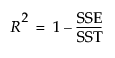where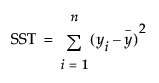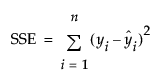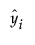are the one-step-ahead forecasts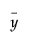is the mean

If the model does not fit the series well, the model error sum of squares, SSE, might be larger than the total sum of squares, SST. As a result, R2 can be negative.

The adjusted R2 value, computed as follows: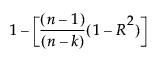MAPE

The Mean Absolute Percentage Error value, computed as follows: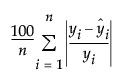whereare the one-step-ahead forecasts

MAE

The Mean Absolute Error value, computed as follows: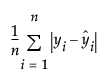whereare the one-step-ahead forecasts

Parameter Estimates

A table that shows the estimate, standard error, and 95% confidence interval for each parameter in the model.

Forecast

A plot that shows both the observed and predicted values for the time series. See Forecast.

A plot of the one-step-ahead forecasting errors over time. For each time point t, the error is calculated as the observed value of the time series at t minus the one-step-ahead predicted value at t. If a holdback set is specified, the errors are computed only for the training data. The autocorrelation and partial autocorrelation reports for these errors are also shown.

Forecasting Errors on Holdback Series

(Available only if a holdback set is specified.) A plot of the forecasting errors on the observations in the holdback set. The autocorrelation and partial autocorrelation reports for these errors are also shown.

Component States

A report that contains a plot for each state of the model and the corresponding formula for the model. Each plot includes the state estimates of the series and the state forecasts. If a holdback set is specified, the state estimates are for the training portion and the state forecasts are for the holdback portion. If a holdback set is not specified, the state estimates are for the portion up to the last observation of the time series and the state forecasts are for after the last observation of the time series. A model can have up to three states: Level, Trend, and Seasonal.

The Component States red triangle menu has a Save States option. Select this option to save the component states at each time point to a separate data table.

One-Step-Ahead Relative Forecasting Errors for Multiplicative Error Model

(Available only for multiplicative error models.) A plot of the one-step-ahead relative forecasting errors over time. For each time point t, the error is calculated as the one-step-ahead-forecasting error at t divided by the one-step-ahead predicted value at t.

### State Space Smoothing Report Options

Each state space smoothing report has a red triangle menu that contains the following options:

Show Points

Shows or hides the data points in the forecast graph.

Show Prediction Interval

Shows or hides the prediction intervals in the forecast graph.

Save Columns

Creates a new data table that contains columns that represent the results of the model.

Remove Fit

Removes the individual model fit from the report.